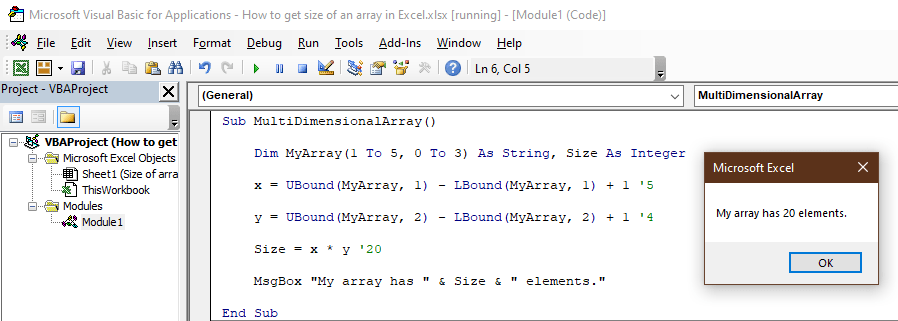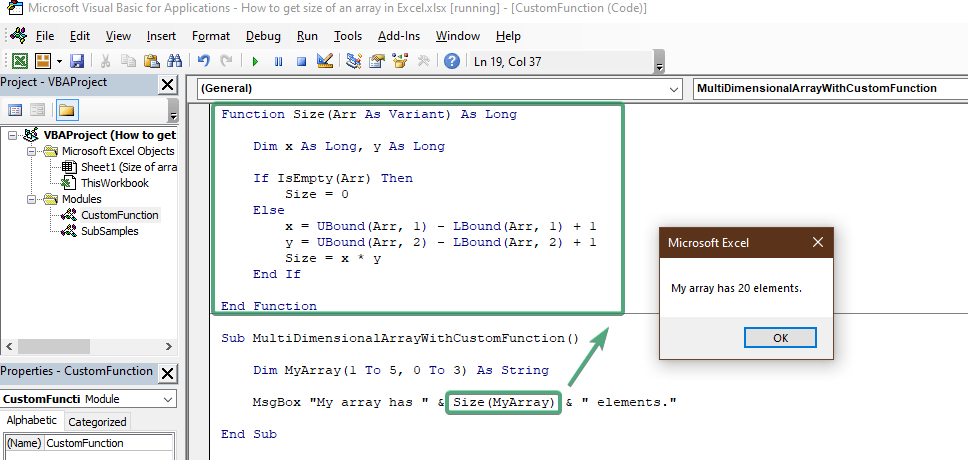An array is a collection of similar-type values or objects in computer science. This isn’t different for Excel. The only caveat is that Excel has two definitions for arrays, one for workbooks and the other for VBA. In this guide, we’re going to show you How to get the size of an array in Excel for VBA.

## How to get size of an array in VBA

In VBA, an array is a variable that can store multiple variables. Although VBA doesn’t have a dedicated function to get the size of an array directly, it has UBound and LBound functions to return the upper and lower limits of a given array.

Note: The LBound function is needed because VBA allows you to use a custom index rather than 0. Thus, a VBA array can start from an index of 2.

Each function shares the same syntax which requires the array variable obviously. If your array is a one-dimensional array just supply the array name.

UBound(MyArray)

LBound(MyArray)

For multidimensional arrays, the second argument is necessary to determine which dimension you want.

UBound(MyArray, 1) 'Upper limit of the first dimension of the "MyArray"

LBound(MyArray, 2) 'Lower limit of the second dimension of the "MyArray"

Once the limits are found the math is simple for a one-dimensional array:

Size = UBound(MyArray) - LBound(MyArray) + 1For a multi-dimensional array, you need to multiply the lengths of each dimension:

x = UBound(MyArray, 1) - LBound(MyArray, 1) + 1

y = UBound(MyArray, 2) - LBound(MyArray, 2) + 1

Size = x * y## Custom function to calculate your array's size

If you need to calculate your arrays' size frequently, you can create your custom function to not to repeat the same code block over and over.

All you need to do is to code a function instead of a regular subroutine. The difference between both is ability to return a value. You can think of an Excel function.

Function Size(Arr As Variant) As Long

Dim x As Long, y As Long

If IsEmpty(Arr) Then

Size = 0

Else

x = UBound(Arr, 1) - LBound(Arr, 1) + 1

y = UBound(Arr, 2) - LBound(Arr, 2) + 1

Size = x * y

End If

End Function